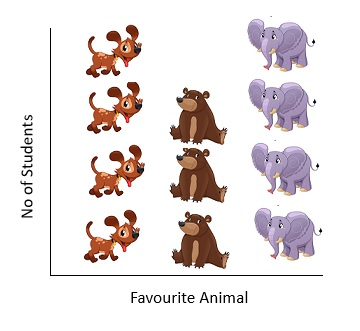# Grade 2, Maths Olympiad (CBSE) - Pictograph

## Pictograph

A pictograph or pictogram represents data using images of the objects. Each image may represent 1 full object or a fraction or multiple of an object. Pictograph are easy to understand and are generally used when the data to be represented is not huge.

E.g.The above fig represents the number of students in a class with their favourite animals.

If we consider as each animal has a magnitude of 1 then dog is the favourite animal of 4 students, bear is the favourite animal of 3 students and elephant is the favourite animal of 4 students.

If we consider as each animal has a magnitude of 5 then dog is the favourite animal of 4 X 5=20 students, bear is the favourite animal of 3 X 5=15 students and elephant is the favourite animal of 4 X 5=20 students.